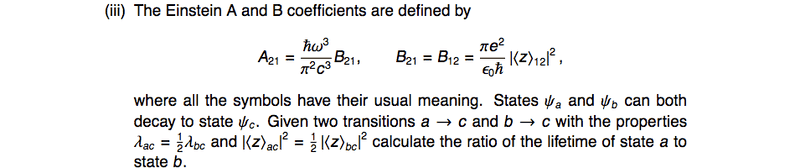# Einstein A & B Coefficients

• bananabandana
In summary, the equations state that the number of atoms in state ##i## is equal to the sum of the atom numbers in states ##A_{ac}+A_{ab}##, where ##A_{ac}## and ##A_{ab}## are the atom numbers in states ##a## and ##b##, respectively. The lifetime is simply the time it takes for the number of atoms in state ##i## to decay by half.f

## Homework Statement## The Attempt at a Solution

Very confused by this problem. For one thing, it doesn't specify if there is or isn't any light present to drive the stimulated emission/absorbtion. I guess there's no reason to assume that there is no light - but since the question is asking about lifetimes, that would seem more sensible...[plus introducing an unspecified ##\rho(\omega_{0})## seems odd]

Assuming there is no light involved, then this is just a three level system, with three sets of coupled differential equations describing the behaviour - let ##N_{i}## be the number of atoms in state ##i##:
(1) $$\frac{dN_{A}}{dt} = -(A_{ac}+A_{ab})N_{A}$$
(2) $$\frac{dN_{B}}{dt} = A_{ab}(N_{A} -N_{B})$$
(3) $$\frac{dN_{C}}{dt} = A_{ac}N_{A}+A_{bc}N_{B}$$
[Though,one of them is made redundant by the fact that total particle number must be constant.]

We can easily solve the first equation:
$$N_{A} = N_{A0}exp\bigg[ - (A_{ac}+A_{ab})t) \bigg]$$
By substituting this into the equation (2), we can then solve for ##N_{B}##:

$$\frac{dN_{B}}{dt} +N_{B}A_{ab} = A_{ab}N_{A0}exp\bigg[ - (A_{ac}+A_{ab})t) \bigg]$$

So by using the standard method of integrating factors:
$$N_{B} = Cexp(-A_{ab}t)-\frac{A_{ab}}{A_{ac}}N_{A0}exp(-A_{ac}+A_{ab}t)$$
But how am I meant to define a lifetime for that?, Assuming ##N_{B}(t=0)=0##, i.e:
$$N_{B} =\frac{A_{ab}}{A_{ac}}N_{A0}exp(-A_{ab}t)\bigg[1-exp(-A_{ac}t)\bigg]$$
Do we take the dominant exponential to define the lifetime?

You are only told to consider the decays a-c and b-c.
The presence of photons is not relevant to the lifetime calculation for a state ... check the definition of "lifetime" in your notes.

You are only told to consider the decays a-c and b-c.
The presence of photons is not relevant to the lifetime calculation for a state ... check the definition of "lifetime" in your notes.

Ah,okay, so lifetimes are generally defined to only involve the Einstein ##A## coefficients.
So I can just ignore completely ##\psi_{A} \rightarrow \psi_{B}##? I wasn't sure the question implied that... I guess if it does:

$$\frac{dN_{A}}{dt} = -A_{ac}N_{A}$$
$$\frac{dN_{B}}{dt} = -A_{bc} N_{B}$$

Implying that ## \lambda_{a} = A_{ac}## ##\lambda_{b} = A_{bc}##. We know that ## \frac{1}{\tau} = \lambda## where ##\tau ## is the lifetime, so:
$$\frac{\tau_{A}}{\tau_{C}} = 2$$

and then the information about the matrix element is completely redundant? It's that simple??
[Edit : I mean, it tells us the same thing]
Thanks!

Well done.

Oh dear, that's embarrassing.. Ah well, many thanks for the help, much appreciated!